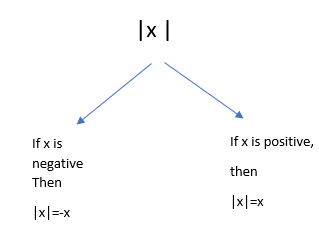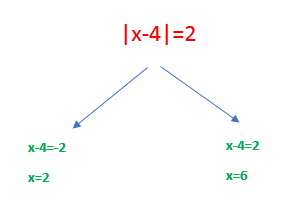# Absolute value equation and Absolute value inequation

## Absolute value equation

Absolute Value is denoted by $|x|$. And it is defined as
$|x|= x$ if $x \geq 0$
$=-x$ if $x < 0$
So it is always positive.Examples
$|x-4|=2$
or $x-4=-2$ or $x-4=2$
or $x=2 \; or \; x =6$Practice Questions
• $\left|{x - 6}\right| = 7$
• $\left|{x - 5}\right| = 9$
• $\left|{x - 6}\right| = 8$
• $\left|{x - 7}\right| = 2$
• $\left|{x - 6}\right| = 7$
• $\left|{x - 2}\right| = 1$
• $\left|{x - 1}\right| = 9$
• $\left|{x - 4}\right| = 1$
• $\left|{x - 1}\right| = 10$
• $\left|{x - 8}\right| = 2$

## Absolute value inequation

$|x-2| > 4$
$|x| < 2$
This is a form of Absolute value inequation
Important Formula's
for a and r being positive real number
• $|x| < a$ implies that $-a< x< a$
• $|x| > a$ implies that $x< -a \; or \; x> a$
• |x|   $\geq$  a implies that x $\geq$ a  or x $\leq$ a
• $|x-a| < r$ implies that  $a-r < x < a+r$
• $|x-a| > r$ implies that  $x < a-r \; or \; x > a+r$
• $a< |x| < b$ implies that x lies in (-b,-a) or (a,b)
• $a< |x-c| < b$ implies that x lies in (-b+c,-a+c) or (a+c,b+c)

## Solved Examples

Question 1
$|x-2| > 4$
Solution
we know from the Formula
$|x-a| > r$ implies that $x < a-r \; or \; x > a+r$
So $x < -2 \; or \; x > 6$
Question 2
$|x| < 2$
Solution:
We know that Formula
|x| < a  implies that   -a< x< a
So  -2 < x < 2
Question 3
$1< |x-2| < 4$
Solution:
We know that Formula
$a< |x-c| < b$ implies that x lies in (-b+c,-a+c) or (a+c,b+c)
So x lies (-4+2,-1+2) or (1+2,4+2)
or x lies (-2,1) or (3,6)